## Example Questions

← Previous 1 3

### Example Question #1 : How To Find Order Of Operations

5 * (482 – 82) + 100 / (3 + 2)2  = ?

66.7

21,746

2004

2400

2016

2004

Explanation:

Order of operations: "PEMDAS” or "Please Excuse My Dear Aunt Sally"

"Parentheses, Exponents, Multiplication and Division, and Addition and Subtraction".

5 * (482 – 82) + 100 / (3 + 2)2  = ?

5 * (400) + 100 / (5) 2  =

2000 + 100 / 25 =

2000 + 4 = 2004

### Example Question #1 : How To Find Order Of Operations

Evaluate:

–1 + 2 * –3

3

7

5

–7

–3

–7

Explanation:

First you perform multiplication 2 * –3 = –6

Then you add –1 which gives you -7.

### Example Question #2 : How To Find Order Of Operations

Evaluate:

10 – 11 * (–1)2

–21

0

1

–1

21

–1

Explanation:

Evaluate the exponent, then distribute, then add.

### Example Question #1 : Order Of Operations

Evaluate the expression:

(2 + 2)– 1

4

7

16

15

1

15

Explanation:

First you perform the operation in the parentheses and then you multiply the exponent; after that, subtract one.

### Example Question #2 : Arithmetic

Evaluate:

(–1) + (2)2 * (–3)

–3

9

–9

13

–13

–13

Explanation:

First you evaluate the exponent. Then you multiply it by (–3) which gives you –12. Add –1 makes it –13.

### Example Question #6 : Order Of Operations

Solve the following equation:

(9 + 1) * (42 + 2) * (72 + 1) / 2 = ?

1500

4500

39

9000

1014.5

4500

Explanation:

Order of operations: "PEMDAS” or "Please Excuse My Dear Aunt Sally"

"Parentheses, Exponents, Multiplication and Division, and Addition and Subtraction".

(9 + 1) * (42 + 2) * (72 + 1) / 2 =

(10) * (16 + 2) * (49 + 1) / 2 =

(10) * (18) * (50) / 2 =

9000/2 = 4500

### Example Question #1 : Order Of Operations

Solve the following equation:

(4 * 12) / (5 + 6 + 1) + 72 + (2 * 1 + 2)3 = ?

90.6

140

126

86

98.6

140

Explanation:

Order of operations: "PEMDAS” or "Please Excuse My Dear Aunt Sally"

"Parentheses, Exponents, Multiplication and Division, and Addition and Subtraction".

(4 * 12) / (5 + 6 + 1) + 72 + (2 * 1 + 2)3 =

(48)/(12) + 72 + (2 + 2)3 =

(48)/(12) + 72 + (4) 3 =

(48)/(12) + 72 + 64 =

4 + 72 + 64 = 140

### Example Question #8 : Order Of Operations

Solve: 4 + 2 * 4 = ?

10

12

32

24

14

12

Explanation:

To solve you must use the order of operations PEMDAS.
Multiplication comes first 4 * 2 = 8. Then add 4 + 8 = 12.

### Example Question #2 : How To Find Order Of Operations

The operation ¤ is defined as “cube the number that is to the right of the ¤ and subtract the result from the number that is to the left of ¤.” What is the value of 100 ¤  (5 ¤ 1)?

25

64

86

16

36

36

Explanation:

Starting inside the parentheses, cube the number to the right of the symbol, which is 1, and then subtract that from the number to the left of the symbol, 5, to get 4. Then we perform the same operation by cubing 4 to get 64, and then subtracting that from 100 to get 36.

### Example Question #1 : Order Of Operations

The expression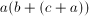is equivalent to: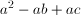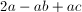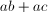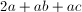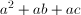In order to find the equivalent expression, distributeto get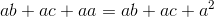.